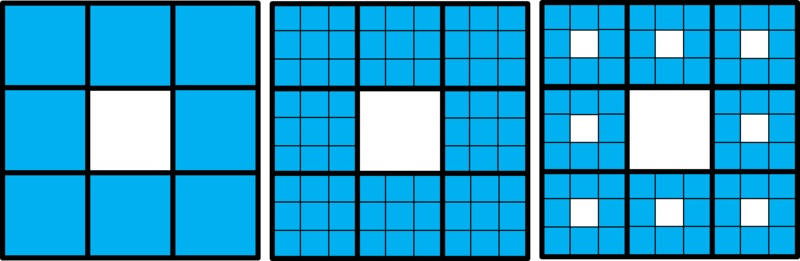# Sierpinski's Carpet

Alignments to Content Standards: 6.G.A.1 6.EE.A.1

1. Take a square with area 1. Divide it into 9 equal-sized squares. Remove the middle one.What is the area of the figure now?

2. Take the remaining 8 squares. Divide each one into 9 equal squares. Remove the middle one from each group of 9.What is the area of the figure now?

3. Take the remaining squares. (How many are there?) Divide each one into 9 equal squares. Remove the middle one from each group of 9.What is the area of the figure now?

4. Imagine you follow this same process until you have removed "the middle square from each group of 9" 10 times. How many squares will there be? What will the area of each little square be? What will the area of the entire figure be?

## IM Commentary

The purpose of this task is to help motivate the usefulness of exponential notation in a geometric context and to give students an opportunity to see that sometimes it is easier to write a number as a numeric expression rather than evaluating the expression, which is an important facet of MP7, Look for and make use of structure. The task assumes that students have seen and evaluated numeric expressions that involve whole-number exponents. Even so, students might not think to use exponential notation to represent the areas. If they haven't, part (d) will be very challenging. Because students are most likely to find an answer to part (d) by noting that at each step all squares are replaced by 8 squares that are $\frac19$ as big, this task naturally engages students in MP8, Look for and express regularity in repeated reasoning.

Students might not realize that the last figure is the same size as the others, just zoomed in to better see the details. Given the complexity of the task, this would be a good activity for students to work on in groups. Another solution approach students might take is to subtract the "holes" left by removing squares from 1, and it would be instructive for students who approach this task in different ways to compare their solutions to verify they give the same results.

The figures students are generating at each step are the figures whose limit is called "Sierpinski's carpet." This is a fractal whose area is 0 and perimeter is infinite! A very challenging extension is to ask students to find the perimeter of each figure in the task. Note that the perimeter includes the lengths of the edges of the "holes" that are left by removing the "middle square in each group of 9." For example, the perimeter of the figure in the third step is $$4+\frac43+8\cdot\frac49+64\cdot\frac{4}{27}$$ The mathematics required to figure out the perimeter of the 10th step is technically accessible to 6th graders, but the complexity of the reasoning means this would be very time-consuming. If students want to learn more about fractals, there are many interesting websites; here is one: http://fractalfoundation.org/

## Solution

1. When we divide the square into 9 smaller, equal-sized squares, each new (smaller) square will have area $\frac19$. If we remove one, there will be 8 left. So the area of the figure is $$\frac89$$

2. When we divide the smaller squares each into 9 new (even smaller), equal-sized squares, each of the new squares will have area $\frac19$ of the area of the square it was made from (which also happens to be $\frac19$). So the area of these even smaller squares is $\frac19\cdot\frac19=\frac{1}{9^2}=\frac{1}{81}$. There are $8\cdot8=8^2=64$ of these squares (one factor of 8 is the number of squares being subdivided and the other factor of 8 is the number of new squares these are divided into). So the area of the figure is $$\frac{8^2}{9^2}=\frac{64}{81}$$

3. When we divide each square of the figure in part (b) into 9 smaller, equal-sized squares, each new square will have area $\frac19$ of the area the square it was made from, which is $\frac{1}{9^2}$. So the area of these even smaller squares is $\frac19\cdot\frac{1}{9^2}=\frac{1}{9^3}=\frac{1}{729}$. There are $8\cdot8\cdot8=8^3 = 512$ of these squares ($8 \cdot 8$ is the number of squares being subdivided and the other factor of 8 is the number of new squares these are divided into). So the area of the figure is $$\frac{8^3}{9^3}=\frac{512}{729}$$

4. Every time we divide a square into 9 equal squares and remove the middle one, we get 8 times as many squares as we had before, but their length and width are $\frac13$ as big, so their area is $\frac19$ as big. This means that the area from the previous step is multiplied by $\frac89$. It is easier to keep track of all of this in a table:
Iteration number Number of squares Size of the squares Total area
Step 0 1 1 1
Step 1 8 $\frac19$ $\frac89$
Step 2 $8^2$ $\frac{1}{9^2}$ $\frac{8^2}{9^2}$
Step 3 $8^3$ $\frac{1}{9^3}$ $\frac{8^3}{9^3}$
Looking at the table, we can see that in the "$n^{\text{th}}$" step, the area will be $\frac{8^n}{9^n}$. So after doing this process 10 times, the area will be $\frac{8^{10}}{9^{10}}\approx 0.31$.